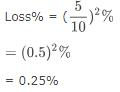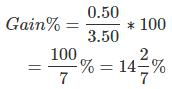Courses

# Test: Profit And Loss- 2

## 10 Questions MCQ Test General Aptitude for GATE 2020 | Test: Profit And Loss- 2

Description
This mock test of Test: Profit And Loss- 2 for Quant helps you for every Quant entrance exam. This contains 10 Multiple Choice Questions for Quant Test: Profit And Loss- 2 (mcq) to study with solutions a complete question bank. The solved questions answers in this Test: Profit And Loss- 2 quiz give you a good mix of easy questions and tough questions. Quant students definitely take this Test: Profit And Loss- 2 exercise for a better result in the exam. You can find other Test: Profit And Loss- 2 extra questions, long questions & short questions for Quant on EduRev as well by searching above.
QUESTION: 1

Solution:
QUESTION: 2

### A man sells two houses at the rate of Rs.1.995 lakhs each. On one he gains 5% and on the other, he loses 5%. What is his gain or loss percent in the whole transaction?

Solution:

The correct answer is A asQUESTION: 3

### A trader gives 12% additional discount on the discounted price, after giving an initial discount of 20% on the labeled price of an item. The final sale price of the item is Rs.704. Find out the labeled price?

Solution:

Let the marked price be Rs. 100.
Successive discounts of 20% and 12% were given.
Sale price = 100 × 80/100 × 88/100 = Rs.704/10
If the sale price is Rs.704/10, the marked price is Rs.100
If the sale price is Rs.704, the marked price is
= 100 × 10 × 704/704 =  Rs.1000

QUESTION: 4

On selling 17 balls at Rs. 720, there is a loss equal to the cost price of 5 balls. What is the cost price of a ball?

Solution:

Let the cost price of a ball be Rs. x.

So, the CP of 17 balls is Rs. 17x.

The CP of 5 balls is Rs. 5x.

Since Loss = CP - SP

So, 5x = 17x - 720

On solving, we get

12 x = 720

that is, x = 60

So, the cost price of a ball is Rs. 60.

QUESTION: 5

If a material is sold for Rs.34.80, there is a loss of 25%. Find out the cost price of the material?

Solution:

The correct answer is A as
cost price
=34.80×100/75
=46.40

QUESTION: 6

A shopkeeper sells one more radio for Rs. 840 at a gain of 20% and another for Rs. 960 at a loss of 4%. What is his total gain or loss percentage?

Solution:

Case 1) S.P of transistor = Rs 840
Profit = 20%
By unitary method C.P = (100/120) ×840= 700Rs
Case 2) S.P of transistor = Rs960
Loss = 4%
By unitary method C.P = (100/96) × 960 = 1000 Rs
Total C.P = 700 + 1000 =1700 Rs
Total S.P= 840 +960 = 1800Rs
There is a profit of = 1800 - 1700 = Rs100
Therefore profit% = (100/1700) × 100 = 100/17
= 5.882% Ans

QUESTION: 7

A fruit seller sells apples at the rate of Rs.9 per kg and thereby loses 20%. At what price per kg, he should have sold them to make a profit of 5%?

Solution:
QUESTION: 8

John purchased a machine for Rs. 80,000. After spending Rs.5000 on repair and Rs.1000 on transport he sold it with 25% profit. What price did he sell the machine?

Solution:

C.P. = (80000 + 5000 + 1000)
⇒ 86000
As we know that Profit = 25%
S.P. = 125% of 86000
⇒ (125/100 * 86000)
⇒ 107500 Rs.

QUESTION: 9

100 oranges were brought at the rate of Rs. 350 and sold at the rate of Rs. 48 per dozen. What is the percentage of profit or loss?

Solution:

So before solving this question we will get the C.P. and S.P. of 1 article to get the gain percent.

C.P. of 1 orange = 350/100 = Rs 3.50
S.P. of one orange = 48/12 = Rs 4 [note: divided by 12 as 1 dozen contains 12 items]
Gain = 4 - 3.50 = Rs 0.50Profit

QUESTION: 10

When an item is sold for Rs. 18,700, the owner loses 15%. At what price should that plot be sold to get a gain of 15%?

Solution:

85 : 18700 = 115 : x
⇒ x = (18700*115)/85
x = 25300